# Hexaprism container

Calculate the volume and surface in the shape of a regular hexagonal prism with a height of 1.4 m with a base edge of 3dm and a corresponding height of 2.6 dm.

V =  327.6 dm3
S =  298.8 dm2

### Step-by-step explanation: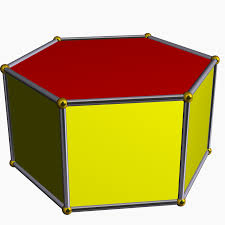Did you find an error or inaccuracy? Feel free to write us. Thank you!Tips to related online calculators
Tip: Our volume units converter will help you with the conversion of volume units.

#### You need to know the following knowledge to solve this word math problem:

We encourage you to watch this tutorial video on this math problem:

## Related math problems and questions:

• Hexagonal prism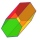Calculate the volume and surface of a regular hexagonal prism with the edge of the base a = 6 cm with the corresponding height v1 = 5.2cm and the height of the prism h = 1 dm.
• Hexagonal pyramidRegular hexagonal pyramid has dimensions: length edge of the base a = 1.8 dm and the height of the pyramid = 2.4 dm. Calculate the surface area and volume of a pyramid.
• Hexagonal pyramidCalculate the volume and surface area of a regular hexagonal pyramid with a base edge a = 30 m and a side edge b = 50 m.
• Triangular prismThe regular triangular prism has a base edge of 8.6 dm and a height of 1.5 m. Finf its volume and surface area.
• Hexagon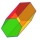Calculate the regular hexagonal prism's surface whose base edge a = 12cm and side edge b = 3 dm.
• A regular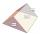A regular triangular prism with a base edge of 20 dm and a height of 30 dm is drawn. Find the volume of the prism and the area of the shell.
• Hexagonal prismThe base of the prism is a regular hexagon consisting of six triangles with side a = 12 cm and height va = 10.4 cm. The prism height is 5 cm. Find the volume and surface of the prism.
• Regular triangular prismCalculate the surface area of body of regular triangular prism, when the length of its base edge is 6.5 cm and height 0.2 m.
• Triangular prismCalculate the volume and surface area of a triangular prism if it is given: a = 6.8 dm. ..Va = 4 dm. (base edge length and base triangle height length) ... ... .v = 23 dm (body height)
• Hexagonal prism 2The regular hexagonal prism has a surface of 140 cm2 and height of 5 cm. Calculate its volume.
• Hexagonal pyramidCalculate the volume and the surface of a regular hexagonal pyramid with a base edge length of 3 cm and a height of 5 cm.Calculate the surface of a quadrilateral prism according to the input: Area of the diamond base S1 = 2.8 m2, length of the base edge a = 14 dm, height of the prism 1,500 mm.Find the volume and surface area of prism with base of an equilateral triangle with side 7 dm long and the body height of 1.5 m.Right-angled prism, whose base is a right triangle with leg a = 3 cm and hypotenuse c = 13 cm, has the same volume as a cube with an edge length of 3 dm. a) Find the height of the prism b) Calculate the surface of the prism c) What percentage of the cube'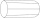The tank has the shape of a regular octagonal prism without an upper base. The base edge has a = 3m, the side edge b = 6m. How much metal sheet is needed to build the tank? Do not think about losses or sheet thickness.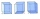Calculate the surface of a regular quadrilateral prism whose base edge is 2.4dm and the height of the prism is 38cm.Calculate the height of the prism having a surface area 448.88 dm² wherein the base is square with a side of 6.2 dm. What will be its volume in hectoliters?